PHY_10_37_V2_LP_Capacitors
Оценка 4.7

# PHY_10_37_V2_LP_Capacitors

Оценка 4.7
docx
07.05.2020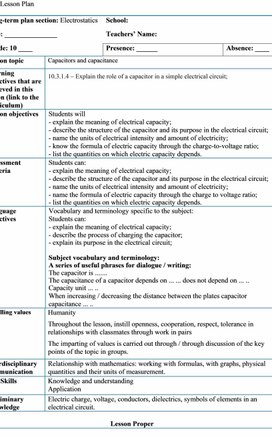PHY_10_37_V2_LP_Capacitors.docx

Lesson Plan

Long-term plan section: Electrostatics

School:

Date: _______________

Teachers’ Name:

Presence: ______

Absence: ____

Lesson topic

Capacitors and capacitance

Learning objectives that are achieved in this lesson (link to the curriculum)

10.3.1.4  Explain the role of a capacitor in a simple electrical circuit;

Lesson objectives

Students will

- explain the meaning of electrical capacity;

- describe the structure of the capacitor and its purpose in the electrical circuit;

- name the units of electrical intensity and amount of electricity;

- know the formula of electric capacity through the charge-to-voltage ratio;

- list the quantities on which electric capacity depends.

Assessment Criteria

Students can:

- explain the meaning of electrical capacity;

- describe the structure of the capacitor and its purpose in the electrical circuit;

- name the units of electrical intensity and amount of electricity;

- name the formula of electric capacity through the charge to voltage ratio;

- list the quantities on which electric capacity depends.

Language objectives

Vocabulary and terminology specific to the subject:

Students can:

- explain the meaning of electrical capacity;

- describe the process of charging the capacitor;

- explain its purpose in the electrical circuit;

Subject vocabulary and terminology:

A series of useful phrases for dialogue / writing:

The capacitor is .......

The capacitance of a capacitor depends on ... ... does not depend on ... ..

Capacity unit ... ..

When increasing / decreasing the distance between the plates capacitor capacitance ... ..

Instilling values

Humanity

Throughout the lesson, instill openness, cooperation, respect, tolerance in relationships with classmates through work in pairs

The imparting of values is carried out through / through discussion of the key points of the topic in groups.

Interdisciplinary communication

Relationship with mathematics: working with formulas, with graphs, physical quantities and their units of measurement.

Key Skills

Knowledge and understanding

Application

Preliminary knowledge

Electric charge, voltage, conductors, dielectrics, symbols of elements in an electrical circuit.

Lesson Proper

Scheduled Lesson Stages

Scheduled Lesson Activities

Resources

Beginning

10 min

At the beginning of the lesson, after the greeting, the teacher invites the students to consider the demonstration set assembled on his desk. Teacher invites two volunteer come to the board, and ask to draw a circuit diagram of an electrical circuit, for the circuit.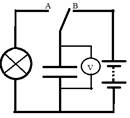Teacher describes the circuit and students draw a circuit diagram.

Circuit is checked by the teacher and whole class discussion takes place.

Teacher discuss with students the following questions:

1. What are the elements included in the electrical circuit?

2. In what position should the switch be in order for an electric current to flow in the circuit?

3. Can there be a current in the circuit if the switch is transferred from position A to position B?

After discussion, teacher demonstrates this circuit and the graph of voltage versus time on the board, while the switch is in position B.

Then switch is in the position B, and students observe the process of discharging the capacitor through the lamp.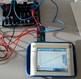Invite students to discuss the role that element X plays in the circuit.

Students during the discussion may hypothesize that it seems to transfer energy from one part of the circuit to another. His role is to store energy and then give it away.

There may be students who call this element a capacitor.

Teacher introduces students topic of the lesson, discuss with them learning objectives and the criteria for evaluating a lesson.

Teacher also introduces the lesson plan to students:

1. Watch and discuss the video.

2. Study of the relationship between the charge of a capacitor and the voltage between its plates.

Laboratory setup: power source, light bulb, capacitor, voltmeter, key, connecting wires

Middle

5 min

Watch the video which presents the process of charging the capacitor (up to 1:39 seconds)

Before watching a video, he introduces students to questions they need to answer:

1. List the main parts of the capacitor.

2. What happens to the charge distribution on the plates while charging the capacitor?

3. How does the speed of movement of electrons between the plates over time?

4. What can be said about the charge that the capacitor plates receive during charging?

After watching the video, students answer the questions.

10 min

7 min

Next, the teacher discusses with them the questions:

1. Will there be an electric field between the capacitor plates?

2. How is its intensity related to the amount of charge on the plates?

3. How is the voltage between the plates related to the field strength, the charge of the capacitor?

Return to the schedule obtained at the beginning of the lesson while charging the capacitor. The teacher suggests comparing the graph shown in the q (t) and U (t) video obtained during the experiment.

From the comparison it becomes clear that the charge and voltage change equally over time. I.e q ~U.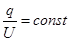To bring students at a qualitative level to an understanding of the relationship

Next, teacher ask student work on virtual lab and check this ratio.

Students work in a small groups. Task of each group is investigate the different parameters by varying the area of the plates and the distance between them.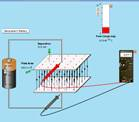Determination of the capacitor charge to the potential difference between the capacitor plates.

Using the options to change the distance between the plates and increase the area of the plates set the following parameters for the capacitor:

Group 1 d = 5 mm and S = 400 mm2

Group 2 d = 10 mm and S = 400 mm2

Group 3 d = 5 mm and S = 200 mm2

Group 4 d = 10 mm and S = 200 mm2

Group 5 d = 5 mm and S = 300 mm2

6 group d = 10 mm and S = 300 mm2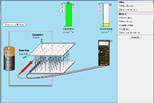1.  In the right part of the window in the “Meters” panel, select the checkboxes on the “Plate charge”, “Voltmeter” and “Capacitance” switches.

2.  Connect the voltmeter to the capacitor as shown in the figure.

3.  Set the slider on the battery to zero position.

4.  Slowly move up and write down the charge values and the volmeter readings for 4 positions in the table.

5.  Calculate the ratio of charge to potential difference between the plates (the values are slightly different, but approximately the same).

6.  Compare the recorded values with the “Capacitance” readings.

Data Table

 № Capacitor charge / C Potential difference between the plates / V The ratio of charge to the potential difference between the plates / C/V Sensor readings Capacitance / F 1 2 3 4 5 6

Teacher summarizes the results of students on the blackboard in the table:

 № Distance m / y plates, mm Plate area, mm2 The average value of the ratio of the charge to the potential difference between the plates / C/V 1 2 3 4 5 6

The teacher discusses with students the results obtained and focuses the students on the following issues:

1. What can be said about the ratio of charge to potential difference in each of the cases considered?

2. How can one explain the slight discrepancy in the results obtained?

3. Does this ratio depend on the charge and the potential difference between the capacitor plates?

4. Does this ratio depend on the distance between the plates? Square plates?

Here they will compare the original data and the results of the groups. Most students can see that this ratio depends on the distance between the plates and their area.

Some students can trace the patterns: a) the greater the distance, the smaller the value of the relationship; b) the larger the area of the plates, the greater this ratio.

The teacher leads students to understand that this relationship characterizes a capacitor and is called capacitance.

The teacher invites students take notes on their copybooks, using a sheet with key concepts and formulas (attached to the lesson):

The electric capacity, a quantity characterizing the ability of a conductor to hold an electrical charge.

- Units of measurement - 1 Farad.

- A capacitor, a device of two or more conductive electrodes (plates), separated by a dielectric, the thickness of which is small compared to the dimensions of the plates, which has a significant electrical capacitance.

- Capacitor charge, the absolute value of the charge of one of the plates.

Basic formulas:

Electric capacity: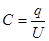hangouts

End

5 min

Assess the level of achievement of the lesson assessment criteria by ticking the appropriate box.

 Criteria I can NOT SURE Still have  difficulty - explain the meaning of electrical capacity; - describe the structure of the capacitor and its purpose in the electrical circuit; - name the units of electrical intensity and amount of electricity; - name the formula of electric capacity through the charge to voltage ratio; - list the quantities on which electric capacity depends.

For the teacher! This table is designed to identify difficulties and the organization of individual work.

H/w Solve the problem

Capacitor charge q = 3 • 10-8 C. The capacitance of the capacitor is C = 10 pF.

(a) Determine the voltage between the capacitor plates.

(c) * Determine the speed that an electron acquires as it flies from one plate to another in a capacitor. The initial electron velocity is zero. Electron specific charge is 1.76*10^11C/kg

Differentiation - how do you plan to provide more support? What tasks do you plan to set for more capable students?

Grading - how do you plan to check students' level of learning?

Health & Safety

Support for students is planned to provide through discussion of the experiment and watching the video. Working in groups with an interactive model will help students visually verify that electrical capacity does not depend on charge and voltage, but depends on the distance between the plates and their area.

Through a discussion of the results of working with an interactive model.

Creating a favorable psychological atmosphere, the compliance of tasks to the level of preparedness of students, the change of activities, counseling.

What tasks do you plan to set for more capable students?

Providing advice to students who have difficulty

Students will:

Most students will:

Some learners will be:

- explain the meaning of electrical capacity;

- describe the structure of the capacitor and its purpose in the electrical circuit;

- name the units of electrical intensity and amount of electricity;

- name the formula of electric capacity through the charge to voltage ratio;

- list the quantities on which electric capacity depends;

- summarize the results and trace patterns.

Lesson reflexion

Use this section to reflect on the lesson. Answer the most important questions about your lesson from the left column.

Were the objectives of the lesson / learning objectives realistic?

Have all students reached the DH?

If not, why not?

Is the differentiation correct in the lesson?

Have the lesson temporal stages been sustained?

What were the deviations from the lesson plan and why?

What are two aspects of the lesson that went well (think about both teaching and learning)?

1:

2:

What could contribute to improving the lesson (think about both teaching and learning)?

1:

2:

What did I discover during the lesson about the class or the achievements / difficulties of individual students, what should I look for in the subsequent lessons?

Скачано с www.znanio.ru

## Next, the teacher discusses with them the questions: 1## Next, the teacher discusses with them the questions: 1## For the teacher! This table is designed to identify difficulties and the organization of individual work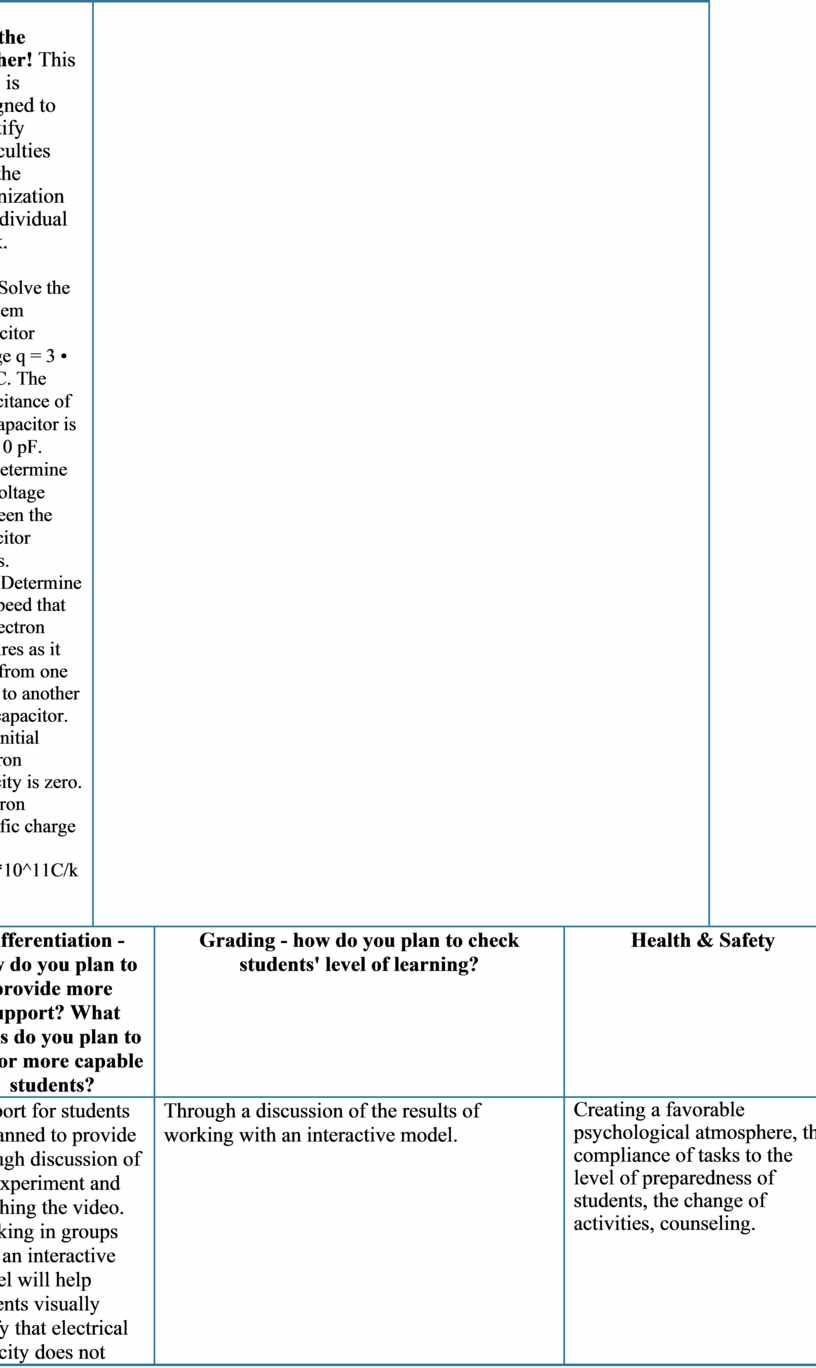Скачать файл